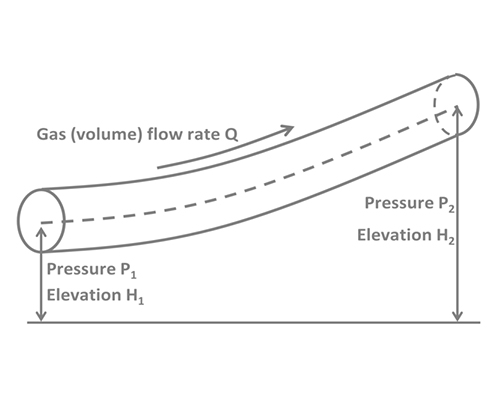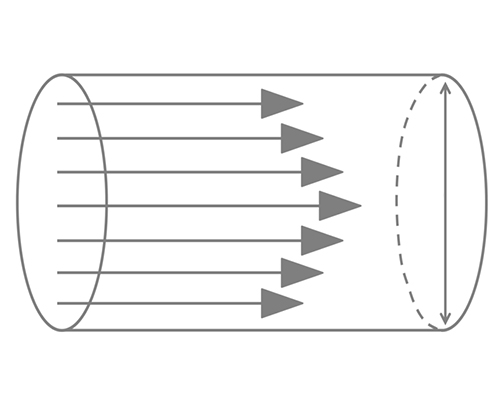Pipeline Design & Analysis – Maple Flow Examples

#Pipeline Design & Analysis – Maple Flow Examples## Flow Rate of Natural Gas Through Pipelines

This application calculates the flowrates of natural gas through a pipeline using several standard equations, including the General Flow Equation, AGA, Weymouth equation, Panhandle A and B equations, and IGT equation.## Pressure Loss and Flow Rates in Pipelines

These predict flowrates and pressure losses in a pipeline by iteratively solving equations that describe the friction factor, head loss and Reynolds number.